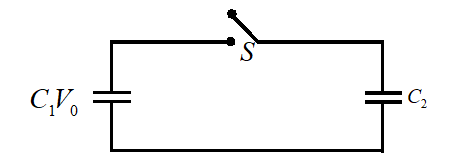A capacitor of capacity C1  is charged to the potential of V0 . On disconnecting from the battery, it is connected with a capacitor of capacity C2 as shown in the adjoining figure. The ratio of energies of system of capacitors before and after the connection of switch  ‘S’ will be

# A capacitor of capacity ${C}_{1}$  is charged to the potential of ${V}_{0}$ . On disconnecting from the battery, it is connected with a capacitor of capacity ${C}_{2}$ as shown in the adjoining figure. The ratio of energies of system of capacitors before and after the connection of switch  ‘$S$’ will be1. A

$\left({C}_{1}+{C}_{2}\right)/{C}_{1}$

2. B

${C}_{1}/\left({C}_{1}+{C}_{2}\right)$

3. C

${C}_{1}{C}_{2}$

4. D

${C}_{1}/{C}_{2}$

Register to Get Free Mock Test and Study Material

+91

Verify OTP Code (required)

### Solution:

Given Capacity of capacitor  $={C}_{1},{C}_{2}$
Potential  ${V}_{1}={V}_{0}$
We know that Common potential  $V=\frac{{C}_{1}{V}_{1}+{C}_{2}{V}_{2}}{{C}_{1}+{C}_{2}}$
Common potential,  $V=\left[\frac{{C}_{1}{V}_{0}+0}{{C}_{1}+{C}_{2}}\right]=\frac{{C}_{1}{V}_{0}}{{C}_{1}+{C}_{2}}$
We know potential energy stored in capacitor,
$U=\frac{1}{2}C{V}^{2}$
Now  $\frac{{U}_{i}}{{U}_{f}}=\frac{\frac{1}{2}{C}_{1}{V}_{0}^{2}}{\frac{1}{2}\left({C}_{1}+{C}_{2}\right){V}^{2}}=\left[\frac{{C}_{1}+{C}_{2}}{{C}_{1}}\right]$
Therefore, the correct answer is (A)Register to Get Free Mock Test and Study Material

+91

Verify OTP Code (required)